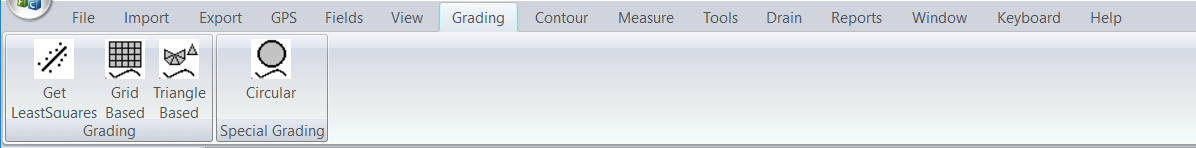This option calculates a number of appropriate parameters applicable to each section. It fills in these values in the appropriate sections in the info bar to the left of Ezigrade.

Also if the min/max grades for grading constraints aren't set then these values are changed to the best fit plane. This is a useful guide if you wish to set the slopes when doing a plane design. You often do this when you wish to match neighbouring planes.

Grading - grid and triangle based

We have supplied two different methods of creating the graded output. They are grid based and triangle based. The legacy method used since day1 is based on gridding the data and working on this. The triangle based method works directly upon the triangles which is a better representation of the base job.

Here are some plus's and minus's of the two different methods:

• grid based allows you to represent a large job by a relatively coarse grid which can give a faster result.
• the grid by definition can lead to loss of features as you are representing the job by a series of discrete points
• the triangle based represents the surface as accurately as we can using the given data.
• the triangle based is going to give a more rigorous result.
• triangle based and large job can give large processing time.
• grading where we make water flow to the edge is only supported using the triangle method.
• maximum cut constraint only works with triangle method.

Clicking on the Grading (grid based)Please note that if you are doing plane design's only then you can simply click OK. Otherwise set a grid size. If you wish to smooth between planes then the smoothing options can be used.

Clicking on the Grading (triangle based)We are given the option of using the existing natural surface as a guide or to use the design surface as a template. So we use the triangles in either the natural or the design surface. If we are starting a new job and we haven't an existing design surface then Ezigrade automatically forces you to use the natural surface as a template. If there is an existing design surface and you have either a drain, outlet or breaklines then Ezigrade will default you to using the design surface as a template. If you have a design surface but no drain etc then Ezigrade will default to using the natural surface triangles.

Here are some relevant thoughts:

• If the graded design surface is only covering part of the job then use the "natural surface".
• If the graded design surface is only covering part of the job but you wish to use existing design breaklines etc. From the Tools menu select the "nat to design" menu item. This will set all the design heights. Now for the design surface. Contours -> Form Model. Now run grading and use design surface as a template.
• If you delete the design menu then it forces Ezigrade to redo the design surface. Click on Contours -> Surface Parameters. Select the design surface and click delete.

Zero grade tolerance. The linear programming routines like a bit of variation. The zero grade tolerance actually says that if min and max grades are the same then allow them to vary plus or minus 0.01%. For more critical applications you can set this to 0.001% for example.

If you are doing planes of best fit then tick the appropriate tick box for either doing a "least squares" solution or alternatively a "linear programming" solution. The least squares solution calculates the plane of best fit by minimising the sum of the least squares difference to all the points in the job. The linear programming solution consists of putting all the points into a mega-big matrix and manipulating it to mimimise a function that approximates the total cut volume.

Or for a non-technical difference. The least squares is quicker but not as accurate. In practice the difference is not that much and probably not worth worrying about.

Smoothing Value.

After the field is graded so that either a plane or constrained grades are produced you have the option of smoothing the surface. This is a bit like running a fine grader over the surface. Smoothing the surface is a good idea as it reduces change of grades and leads to better water run-off.

Many designers simply do the smoothing on appropriate fields. We have been getting positive feedback on this option. Many users prefer this to an alternative method of limiting the change in grade ( for those mathematically inclined - the second differential of the surface).

The smoothing is based on least squares. The "smoothing" distance is how far to look to pick up points to be used in smoothing.

The larger these values are the smoother the surface. If you make the values large in relation to the field then you will basically end up with a plane. Make them both small and there will be little effect.

Here are some sample's from a real job and shows the effect of these values:

When doing a design like this you can trade off the smoothness of the finished surface with the amount of dirt that needs to be removed.

Example1: No smoothing.
Cut Value -10656
Fill Value     8881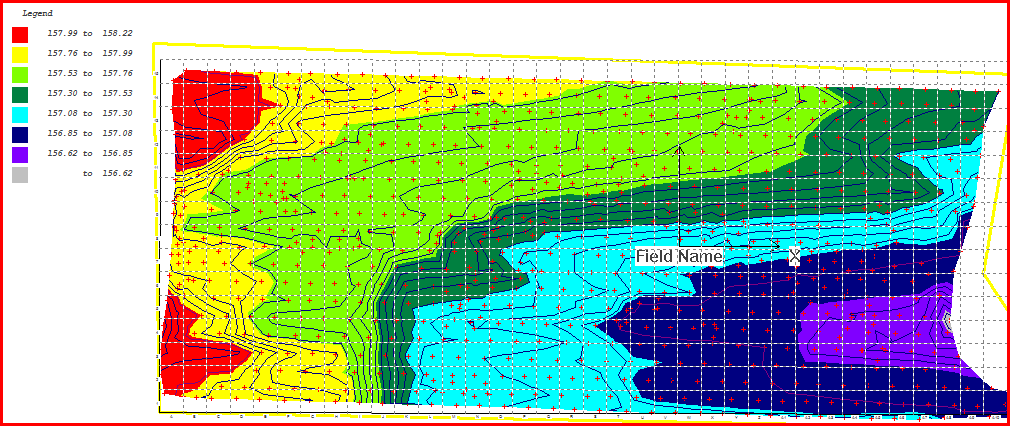Example 2: smoothing values of 30
Cut Value  -11154
Fill Value      9296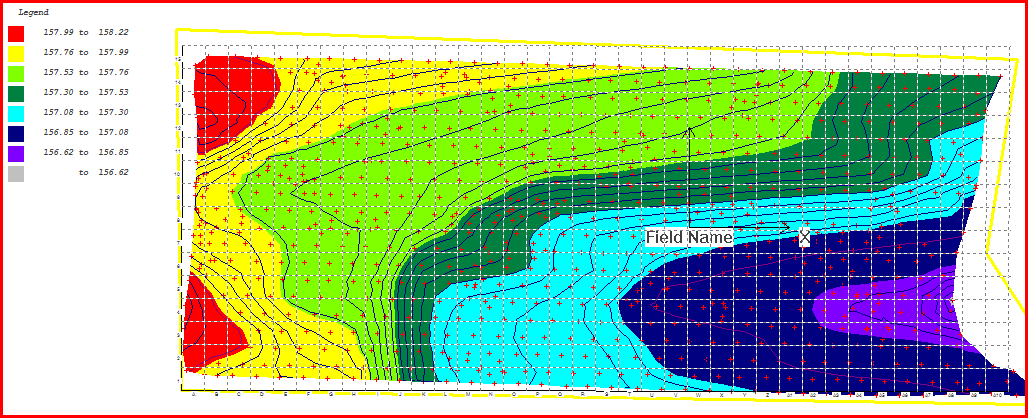Example 3:  smoothing values of 40 and 60 respectively
Cut Value  -12008
Fill Value     10007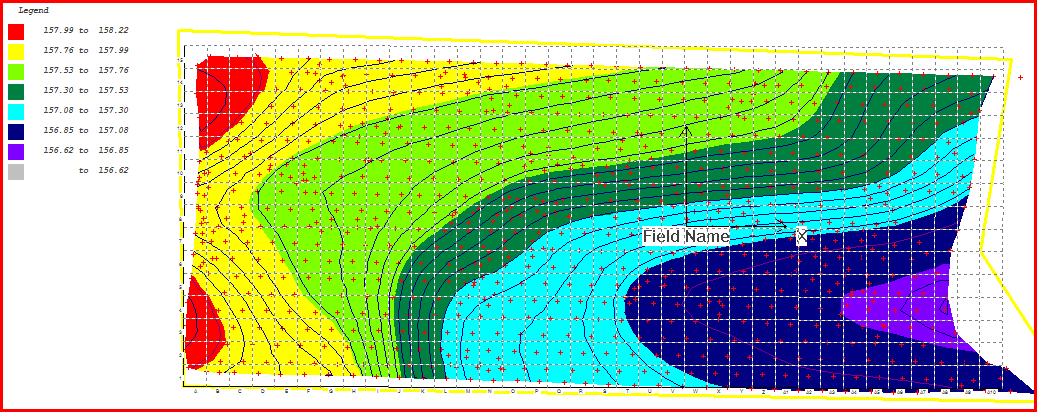Example 4:  smoothing value 120
Cut Value  -13219
Fill Value    11015Example 5:  smoothing value 240
Cut Value  -14400
Fill Value    12001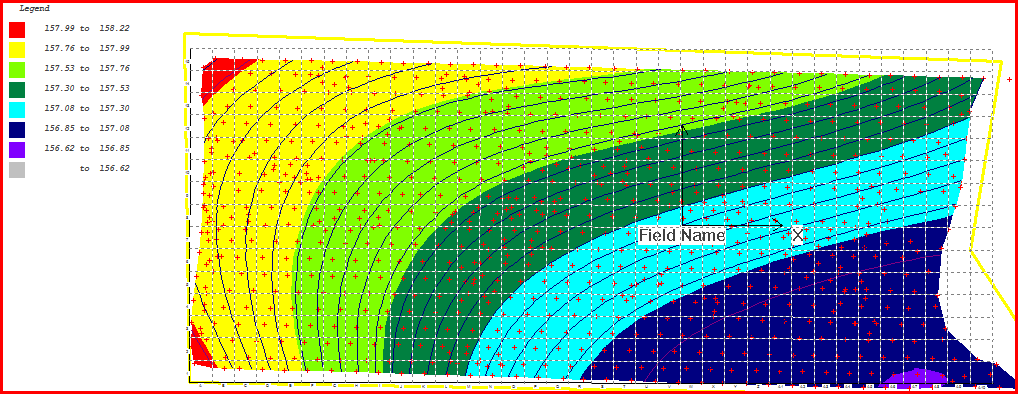TODO: Change the footer's content in Project Settings : HTML (CHM) Export : Setup HTML Template and Layout